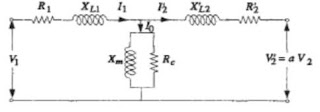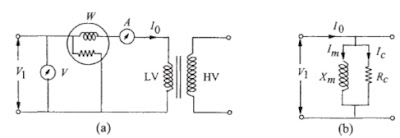Open Circuit and Short Circuit Tests of Transformer

Open circuit test :
Open circuit test determines the parameters of shunt branch of the equivalent circuit of  Fig. 1.
- The rated voltage is applied to one winding and other winding is kept open
- the no-load current is a very small percentage of the full load current, which can be in the range of 0.2 to 2%
- the voltage drop in LV resistance and leakage reactance is negligible as compared to the rated voltage
- The input power measured by a wattmeter consists of the core loss and primary winding ohmic loss.
- If the no-load current is 1% of full load current, ohmic loss in primary winding resistance is just .01% of the load loss at rated current; the value of winding loss is negligible as compared to the core losses. Hence, the entire wattmeter reading can be taken as the total core loss. The equivalent circuit of figure gets simplified to that shown in figure 2.Fig. 1 Transformer equivalent circuitFig. 2  Open circuit test

The no-load (core loss) Pc measured by the wattmeter is expressed as
Pc = V1Io cosθ
From the measured values Pc, V1 and Io of the value of no-load power factor can be calculated from :

With reference to the vector diagram , the magnetizing component (Im)and the core loss component (Ic) of the no-load current (Io ) are

Io = Ic cosθo
Im = Io sinθo

The shunt branch parameters of the equivalent circuit can be estimated as

These values are with reference to the LV side, since the measuring instruments are placed on the LV side. If required, they can be referred to the HV side by using the operator a2. The value of magnetizing reactance is very high as compared to the leakage reactance. For a no-load current of 0.2% and with the assumption that :

since the value of Xm is 500 per-unit.

Short circuit test
A short circuit test is done to measure the load loss and leakage impedance of a transformer.
- In this test, usually the LV winding is short-circuited and voltage is applied to the HV winding in order to circulate the rated currents in both the windings; the voltage required to be applied is called as the impedance voltage of the transformer. For a transformer having 10% leakage impedance, voltage required to circulate the rated current is 10% of the rated voltage.
- The circuit diagram for short circuit test is shown in figure 3.(a), in which LV winding (secondary winding 2) is short-circuited. For an applied voltage of 10%, assuming for the equivalent circuit of figure 1 that the primary and referred secondary leakage impedances are equal, 5% of voltage appears across the shunt excitation branch.
- With a no-load current of 2% at rated voltage, the current in the shunt branch for a 5% voltage is just 0.1% of rated current (assuming linear B-H curve).
- Hence, the shunt branch can be neglected giving the simplified circuit of figure 3.(b) for the short circuit test.
- Since the core loss varies approximately in the square proportion of the applied voltage, with 5% voltage
across the shunt excitation branch, it is just 0.25% of the core loss at the rated voltage. Hence, almost the entire loss measured by the wattmeter is the load loss of the transformer.Fig . 3  Short circuit test (with LV terminals short-circuited

- Equivalent circuit parameters  Req1(= R1 +R'2 ), Xeq1(= XL1 + X'L2 ) and Zeq1(= Req1 +j Xeq1) can now be determined from the measured quantities of power (PL ), voltage ( Vsc )voltage and current (Isc ) as :

- Req1 is the equivalent AC resistance referred to the primary (HV) winding and accounts for the losses in DC resistance of windings, eddy losses in windings and stray losses in structural parts.
- It is not practically possible to apportion parts of stray losses to the two windings.
- Hence, if the resistance parameter is required for each winding, it is usually assumed that R1= (1/2)Req1.
- Similarly it is assumed that  XL1 = X'L2 although it is strictly not true. Since the value of % R is much smaller than % Z, practically percentage reactance (% X) is taken to be the same as percentage impedance (% Z). This approximation may not be true for very small distribution transformers.

Related articles :
1.